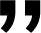# Understanding Pip in Forex Trading: Calculating Value, Profits, and Losses

Article 3 from 8

3 Min

3 Min

4 Min

3 Min

3 Min

4 Min

4 Min

• ### 8. Demo Trading in Forex: From Noob to Pro in No Time!

2 Min

Like the ocean waves, the value of currency pairs in forex is never set in stone. It constantly changes as time goes by.You’ve probably seen or heard about the Dollar weakening or strengthening. Well, those fluctuations in currency values have a direct impact on the exchange rates between different currencies. Understanding the movement of exchange rates is crucial to successful forex trading. Traders can profit from the differences in currency exchange. This is where pip in forex comes into play as a measurement tool in forex trading.

## So, What's a Pip in Forex Anyway?

Pip stands for Percentage in Point. A pip is simply the smallest unit of measurement for price changes in a currency pair. It’s short for “Percentage in Point.” Here’s the deal: 1 pip equals 10 points, or what we call “pipettes.” Usually, you’ll see the value of a pip represented by the fourth decimal place in a currency price. For example, if 1 Euro is valued at 1.0521 USD and it changes to 1.0522 USD, that means it moved by 1 pip. In other words, it went up by 0.0001 USD. But wait, there’s a small exception when it comes to the Japanese Yen (JPY). If a currency is paired with the Yen, then there are only two decimal places involved. So, if the USD to JPY price changes from 101.48 to 101.49, it moved by 1 pip.

## The Smaller Version of Pip: The Pipette

Here is the thing, not all forex brokers follow the exact pip system. Some use pipettes instead. But, don’t worry, it’s pretty much the same concept, just with even smaller decimal increments. For instance, if the price of the US Dollar changes from 1.05214 USD to 1.05215 USD, it moved by 1 pipette. That’s an increase of 0.00001 USD. When it comes to pairs involving the Yen, you look at the third decimal place for pipettes. So, if the USD to JPY price changes from 101.418 to 101.419, it moved by 1 pipette.

## So, How Do You Calculate Pip in Forex?

Before we dive into calculating pips, it’s crucial to know that forex trading is not done with just one dollar. That’s why there are different lot sizes in forex trading, including:

• Standard Contract: 1 lot is equal to 100,000 units (If you’re trading in USD, it means 100,000 USD).
• Mini Contract: 1 lot is equal to 10,000 units (If you’re trading in USD, it means 10,000 USD).
• Micro Contract: 1 lot is equal to 1,000 units (If you’re trading in USD, it means 1,000 USD).

### 1. Calculating Pip Value for Major Pairs

Let’s say you’re trading the USD/CAD pair with an amount of 10,000 USD, and the exchange rate for USD/CAD is 0.9800. You can calculate the pip value using this formula:

Pip Value (Based on Base Currency) = 1 Pip x Exchange Rate

Pip Value = 0.0001 x 0.9800 = 0.000095 USD

This means that if you’re trading with 10,000 USD, a one-pip change in the USD/CAD exchange rate would be equivalent to 0.95 USD (calculated as 10,000 x 0.000095).

Next, you can calculate your profit or loss using the following formula:

Profit or Loss = Number of Pips Gained or Lost x Value of 1 Pip

Let’s assume there’s an increase of 50 pips in your trade. That means:

Profit = 50 pips x 0.95 USD = 47.5 USD.

### 2. Calculating Pip Value for Cross Pairs

Let’s say you’re trading the EUR/GBP pair with an amount of 100,000 Euros, and the exchange rate is 0.8700. You can calculate the pip value using the same formula:

Pip Value (Based on Base Currency) = 1 Pip x Exchange Rate

Pip Value = 0.0001 x 0.8700 = 0.000087 EUR

Since the value is expressed in Euros, let’s convert it to USD. Let’s assume the exchange rate between EUR and USD is 1.06 USD.

Pip Value in USD = Value of 1 Pip in Base Currency x Exchange Rate

Pip Value in USD = 0.000087 x 1.06 = 0.000092 USD

This means that if you’re trading with 100,000 Euros, a one-pip change in the EUR/GBP exchange rate would be equivalent to 8.7 Euros (calculated as 100,000 x 0.000087) or 9.2 USD (calculated as 100,000 x 0.000092).

Next, you can calculate your profit or loss using the following formula:

Profit or Loss = Number of Pips Gained or Lost x Value of 1 Pip

Let’s assume there’s an increase of 50 pips in your trade. That means:

Profit = 50 pips x 9.2 USD = 462 USD.

So, by applying these calculations, you can determine the value of each pip and calculate your potential profits or losses in forex trading. It’s essential to understand these concepts to effectively manage your trades and make informed decisions.

Remember that these calculations of pip in forex may vary depending on your broker and the specific trading platform you use. It’s always a good idea to double-check with your broker or utilize the tools provided by your trading platform to ensure accurate calculations.

#### Article Summary